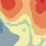# Discrepancies in semivariogram graphs within kriging analysis using the same dataset and specifications in ArcMap and ArcGIS Pro

270
2
a month agoNew Contributor

I am using for most of my research analyses ArcGIS Pro (2.6), but as in it I can't export the semivariogram graph (within the kriging analysis) values, I performed the kriging analysis in ArcMap (10.7.1) to export the semivariogram graph values. Even though I used the same dataset and the exact same specifications (as ordinary kriging, Box-Cox transformation type with no trend) there are discrepancies in the graph x-axis and y-axis. As in the attached pictures can be observed that for the semivariogram graph from ArcGIS Pro the x-axis is "Distance (10^(-1) degrees)" and the y-axis is "y (10^5)". While for the semivariogram graph from ArcMap the x-axis is "Distance (10^(1) degrees)" and the y-axis is "y (10^-5)".  Other than that both graphs and the numbers in the axis are the same no matter if I use ArcGIS Pro or ArcMap.

My concern is that for my analysis is important to know which is the correct order of magnitude from the values of both axes.

I plotted the values from the exported values (exported values are the 1st and 3rd columns, the 2nd and 4th columns are the values adjusted to the order of magnitude in the graph) and also attached a picture of the graph.

1 Solution

Accepted SolutionsbyEsri Regular Contributor

Hi Cristina,

Thank you for your question!  Indeed, the displayed units of the x- and y-axes of the Geostatistical Wizard changed between ArcMap and ArcGIS Pro (specifically, all exponents changed signs).  However, they are both displaying the same information, just using different syntax to display the information.

In ArcGIS Pro, the value in the parentheses is the unit of the axis.  For example, in your semivariogram, a value of 1 on the x-axis means 0.1 (10^-1) decimal degrees as the distance.  On the y-axis, a value of 1 means a semivariance of 100000 (10^5).

In ArcMap, the axes are labeled as "Distance (Unit), h * 10^x".  You should read this as, "The true distance h, when multiplied by 10^x, results in the displayed value on the axis."  For example, with your x-axis, if the true distance were 0.1 decimal degrees, you would multiply it by 10 to get a displayed value of 1 on the axis. This is equivalent to saying, "Multiply the displayed value by 10^-x to get the true value."

While the syntax of the axes used in ArcMap was common in geostatistical research papers, many users (myself included) found this very confusing and would often interpret the magnitude backwards.  We decided to change this in ArcGIS Pro to instead display a more traditional unit.

-Eric

2 RepliesbyEsri Regular Contributor

Hi Cristina,

Thank you for your question!  Indeed, the displayed units of the x- and y-axes of the Geostatistical Wizard changed between ArcMap and ArcGIS Pro (specifically, all exponents changed signs).  However, they are both displaying the same information, just using different syntax to display the information.

In ArcGIS Pro, the value in the parentheses is the unit of the axis.  For example, in your semivariogram, a value of 1 on the x-axis means 0.1 (10^-1) decimal degrees as the distance.  On the y-axis, a value of 1 means a semivariance of 100000 (10^5).

In ArcMap, the axes are labeled as "Distance (Unit), h * 10^x".  You should read this as, "The true distance h, when multiplied by 10^x, results in the displayed value on the axis."  For example, with your x-axis, if the true distance were 0.1 decimal degrees, you would multiply it by 10 to get a displayed value of 1 on the axis. This is equivalent to saying, "Multiply the displayed value by 10^-x to get the true value."

While the syntax of the axes used in ArcMap was common in geostatistical research papers, many users (myself included) found this very confusing and would often interpret the magnitude backwards.  We decided to change this in ArcGIS Pro to instead display a more traditional unit.

-EricNew Contributor

Thank you so much for your quick response!!!